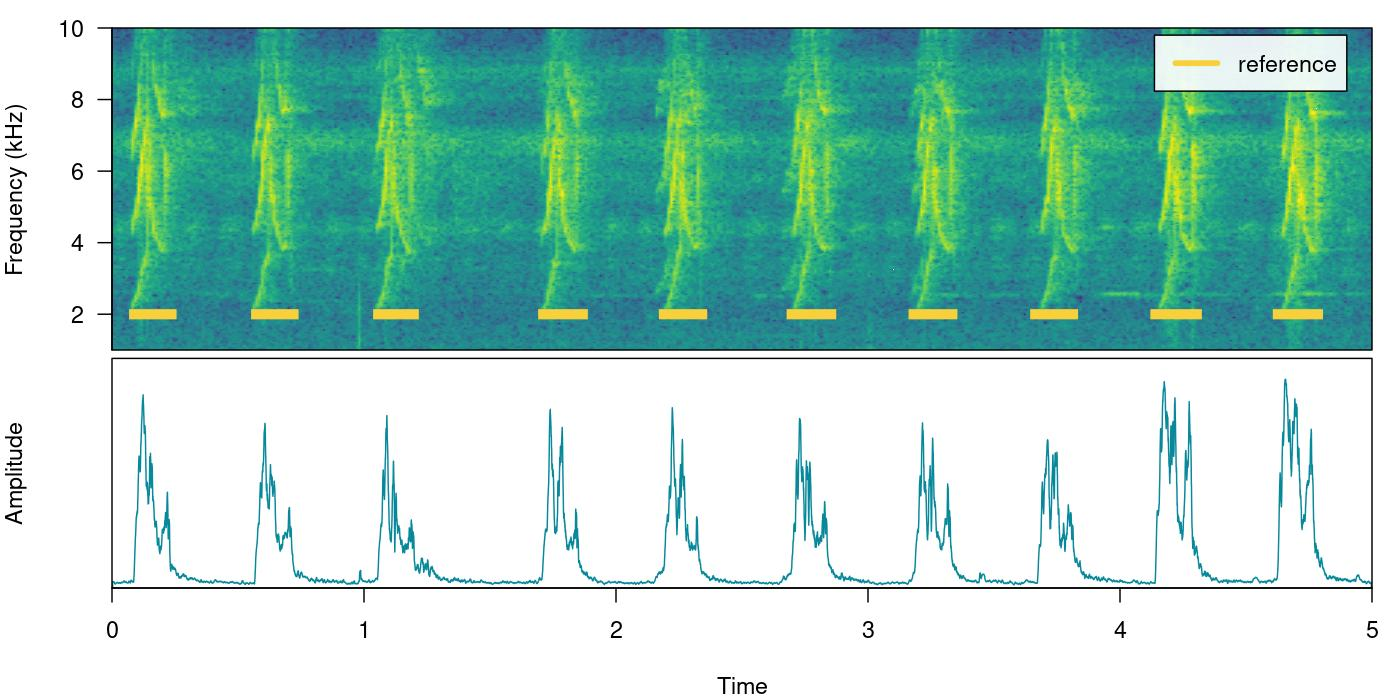This vignette details the use of energy-based detection in ohun. The energy detector approach uses amplitude envelopes to infer the position of sound events. Amplitude envelopes are representations of the variation in energy through time. This type of detector doesn’t require highly stereotyped sound events, although they work better on high quality recordings in which the amplitude of target sound events is higher than the background noise (i.e. high signal-to-noise ratio):

First, we need to install the package. It can be installed from CRAN as follows:

``````# From CRAN would be
install.packages("ohun")

library(ohun)``````

To install the latest developmental version from github you will need the R package remotes:

``````# install package
remotes::install_github("maRce10/ohun")

library(ohun)
library(tuneR)
library(warbleR)``````

The package comes with an example reference table containing annotations of long-billed hermit hummingbird songs from two sound files (also supplied as example data: ‘lbh1’ and ‘lbh2’), which will be used in this vignette. The example data can be load and explored as follows:

``````# load example data
data("lbh1", "lbh2", "lbh_reference")

# save sound files
tuneR::writeWave(lbh1, file.path(tempdir(), "lbh1.wav"))
tuneR::writeWave(lbh2, file.path(tempdir(), "lbh2.wav"))

# select a subset of the data
lbh1_reference <-
lbh_reference[lbh_reference\$sound.files == "lbh1.wav",]

# print data
lbh1_reference``````
``Object of class 'selection_table'``
``* The output of the following call:``
```[.selection_table`(X = lbh_reference, i = lbh_reference\$sound.files == "lbh1.wav")``
``````
Contains: *  A selection table data frame with 10 rows and 6 columns:``````
``|   |sound.files | selec|  start|    end| bottom.freq| top.freq|``
``|:--|:-----------|-----:|------:|------:|-----------:|--------:|``
``|10 |lbh1.wav    |    10| 0.0881| 0.2360|      1.9824|   8.4861|``
``|11 |lbh1.wav    |    11| 0.5723| 0.7202|      2.0520|   9.5295|``
``|12 |lbh1.wav    |    12| 1.0564| 1.1973|      2.0868|   8.4861|``
``|13 |lbh1.wav    |    13| 1.7113| 1.8680|      1.9824|   8.5905|``
``|14 |lbh1.wav    |    14| 2.1902| 2.3417|      2.0520|   8.5209|``
``|15 |lbh1.wav    |    15| 2.6971| 2.8538|      1.9824|   9.2513|``
``... and 4 more row(s)``
``````
* A data frame (check.results) generated by check_sels() (as attribute) ``````
``created by warbleR < 1.1.21``

We can plot the annotations on top of the spectrogram and amplitude envelope to further explore the data (this function only plots one wave object at the time, not really useful for long files):

``````# print spectrogram
label_spectro(wave = lbh1, reference = lbh1_reference, hop.size = 10, ovlp = 50, flim = c(1, 10), envelope = TRUE)``````# How it works

The function `ernergy_detector()` performs this type of detection. We can understand how to use `ernergy_detector()` using simulated sound events. We will do that using the function `simulate_songs()` from warbleR. In this example we simulate a recording with 10 sounds with two different frequency ranges and durations:

``````# install this package first if not installed
# install.packages("Sim.DiffProc")

#Creating vector for duration
durs <- rep(c(0.3, 1), 5)

#Creating simulated song
set.seed(12)
simulated_1 <-
warbleR::simulate_songs(
n = 10,
durs = durs,
freqs = 5,
sig2 = 0.01,
gaps = 0.5,
harms = 1,
bgn = 0.1,
path = tempdir(),
file.name = "simulated_1",
selec.table = TRUE,
shape = "cos",
fin = 0.3,
fout = 0.35,
samp.rate = 18
)\$wave``````

The function call saves a ‘.wav’ sound file in a temporary directory (`tempdir()`) and also returns a `wave` object in the R environment. This outputs will be used to run energy-based detection and creating plots, respectively. This is how the spectrogram and amplitude envelope of the simulated recording look like:

``````# plot spectrogram and envelope
label_spectro(wave = simulated_1,
env = TRUE,
fastdisp = TRUE)``````